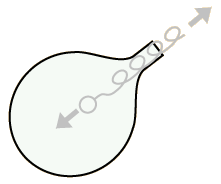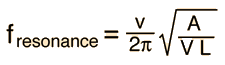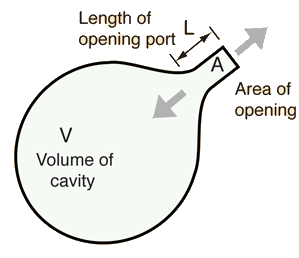# Cavity Resonance

An air cavity will exhibit a single resonant frequency. If extra air is pushed into the volume and then released, the pressure will drive it out. But, acting somewhat like a mass on a spring which is pulled down and then released, it will overshoot and produce a slight vacuum in the cavity. The air will oscillate into and out of the container for a few cycles at a natural frequency. The qualitative nature of the frequency determining factors:Actually the frequency depends upon the square root of these factors and also upon the speed of sound, as you can see in the actual calculation of the frequency. But the above illustration shows the physical factors that are involved in determining the resonant frequency.

 Applications Calculation
 Use for hearing a specific frequency
Index

Wave concepts

Resonance concepts

 HyperPhysics***** Sound R Nave
Go Back

# Cavity Oscillations

The single frequency cavity resonance suggests a parallel with the single resonant frequency of a mass on a spring. In fact, the term "acoustic mass" is sometimes used in connection with such oscillations.Thinking of the cavity resonance in terms of an oscillating mass of air can give some intuition about why the physical properties of the cavity affect the resonant frequency as they do. You can visualize the process of pushing extra air into the cavity to produce an overpressure. That overpressure represents stored energy in a way analogous to lifting a mass on a spring above its equilibrium position. If the opening to the cavity is larger, the excess air can escape more rapidly to bring the pressure down to atmospheric, and this leads to a higher cavity resonant frequency. If the neck of the cavity is longer, there is more resistance to the flow of the excess air and the resonant frequency is lowered. If the cavity volume is increased, then it takes a greater excess mass of air to produce a given overpressure, and it therefore takes longer for that excess pressure to be relieved. The larger cavity will have a lower resonant frequency.

Again visualizing the mass on a spring, we know that if we lift it from equilibrium and allow it to fall, it will not stop when it reaches that equilibrium point but will overshoot it and oscillate about equilibrium because the work we did to lift the mass put energy into the elastic system. Likewise, when we have done work to increase the pressure in a cavity, we have given it energy and as the air rushes out, it will overshoot the equilibrium (atmospheric pressure) and produce a slight vacuum in the cavity. This elastic system produces the cavity resonance, but it is highly damped and will not continue to oscillate like a mass on a spring.

Index

Wave concepts

Resonance concepts

 HyperPhysics***** Sound R Nave
Go Back

# Applications of Cavity Resonance

 Coke bottle Vocal resonance Bass reflex speakers Cavity resonance Brass mouthpiece effect Bass traps Air resonance of string instruments
Index

Wave concepts

Resonance concepts

 HyperPhysics***** Sound R Nave
Go Back

# Cavity Resonant Frequency

A quantitative analysis of the cavity resonance gives the frequency expressionClick on the desired quantity in the formula above.
Frequency, area, volume or length may be calculated by clicking on the desired quantity in the above highlighted formula. Data values not entered will default to the experimental values for a plastic coke bottle used in an example. All parameters may be changed.A = cm2 = m2 = in2 V = cm3 = m3 = in3 L = cm = m = in The cavity resonant frequency is f = Hz For T= C the sound speed is m/s
After entering data, click on the item you wish to calculate in the active formula above.

Note: This calculation uses the sound speed calculated from a linear approximation that only applies fairly close to room temperature. If you are interested in a resonant frequency for a really hot cavity, then you could calculate a sound speed from the more accurate gas equation and plug it in the sound speed box. The temperature it shows in the temperature box will then not be correct, but when you click on "frequency" you will get a better estimate of the resonant frequency of the hot cavity. (This note was added after someone was measuring cavity resonances at oven temperature and was not getting agreement.)
Index

Wave concepts

Resonance concepts

 HyperPhysics***** Sound R Nave
Go Back